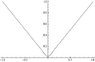# How can I find a polynomial that approximates |x|

• happybear
Legendre polynomials that converge to f in the L^2 norm (mean square). I'm not sure if the two plots are supposed to be correlated or not, I just drew them to illustrate my point. In summary, according to Stone Weierstrass Theorem, there exists a polynomial such that lim P = |x|. Can anyone give me an example of P? Maybe within the range of 0.01?f

## Homework Statement

According to Stone Weierstrass Theorem, there exists a polynomial such that lim P = |x|.
Can anyone give me an example of P? Maybe within the range of 0.01?
ie. |f-P|< 0.01

## The Attempt at a Solution

I'm not sure but I think the Legendre polynomial expansion for |x| would converge uniformly to |x| as stated by Stone-Weierstrass.

I think it converges in the mean. Since the Legendre polynomials are orthogonal, then we can write:

$$f(x)=a_0 P_0(x)+a_1 P_1(x)+a_2 P_2(x)+\cdots$$

with:

$$a_k=\frac{2k+1}{2}\int_{-1}^{1} f(x)P_k(x)dx$$

I'd try say 25 of them programatically (in Mathematica) then compare it to $$f(x)=|x|$$ happybear.

The important facts are that the Legendre polynomials are both orthogonal and complete. This means that the mean square of the difference between f and the first n terms in the series goes to zero as n → ∞, i.e. the series converges uniformly to f.

EDIT: Turns out this is not true. Read jbunniii's post #8.

Last edited:
According to Stone Weierstrass Theorem, there exists a polynomial such that lim P = |x|.
Can anyone give me an example of P? Maybe within the range of 0.01?
ie. |f-P|< 0.01
In the interest of making correct statements, what you mean is that there is a sequence of polynomials {Pn} such that limn -> inf Pn(x) = |x| uniformly in x. (Or... maybe you just mean that it converges at each value of x)

The important facts are that the Legendre polynomials are both orthogonal and complete. This means that the mean square of the difference between f and the first n terms in the series goes to zero as n → ∞, i.e. the series converges uniformly to f.

Mean square convergence does not imply uniform convergence in general!

Consider f_n(x) (for n = 1,2,...) defined on [0,1] as an isosceles triangle whose base is the interval [0,1/n] and whose height is 1. If f(x) = 0 on [0,1] then f_n converges to f in the mean square sense (and also pointwise) but the convergence is not uniform.

Maybe it's true that you can find a sequence of Legendre polynomials that converge uniformly to |x| (I'm not sure one way or the other) but if so, this fact isn't implied by mean square convergence.

P.S. I don't think anyone mentioned it yet, but Stone-Weierstrass applies only on a compact set, so it only applies for f(x) = |x| if you restrict the domain.

P.P.S. The Bernstein polynomials were "used by Bernstein in a constructive proof for the Stone-Weierstrass approximation theorem." -- see http://en.wikipedia.org/wiki/Bernstein_polynomial

Last edited:
The functions in your example are not orthogonal. I could be wrong, but I remember reading somewhere that orthogonality and completeness imply uniform convergence.

The functions in your example are not orthogonal. I could be wrong, but I remember reading somewhere that orthogonality and completeness imply uniform convergence.

Orthogonality and completeness imply L^2 convergence (mean-squared) but not in general L^infinity convergence (uniform). L^2 is special because it is a Hilbert space, whereas L^p is not a Hilbert space if p is not 2.

As I said, I'm not sure whether a sequence of Legendre polynomials that converges to f in L^2 will also converge uniformly. (I haven't found any statements or proofs to that effect after a fair amount of Googling.) But the orthogonality/L^2 convergence alone are not enough to guarantee this.

By the way, there's a very similar situation with Fourier series: the complex exponentials are an orthonormal basis for L^2 and their linear combinations are dense in L^2. For any function f in L^2, its Fourier series converges to f in the L^2 norm (mean-squared sense).

However, there exist even continuous functions whose Fourier series (the partial sums of which are trigonometric polynomials) don't converge at every point (the best you can hope for is pointwise convergence except on a set of measure 0), let alone converge uniformly.

You CAN find a sequence of trigonometric polynomials that do converge uniformly to f if it's continuous [this is in fact also guaranteed by the Stone-Weierstrass theorem if you use a sufficiently general version], but you have to construct them using Fejer sums instead of the "raw" Fourier series.

This is analogous to the situation with Stone-Weierstrass and polynomials - there certainly exist uniformly approximating polynomials to any continuous function on a compact set, but just because you find a sequence of polynomials (orthonormal ones, even) that converges in some other sense (pointwise or L^2) doesn't mean that particular sequence converges uniformly.

Last edited:
I think it converges in the mean. Since the Legendre polynomials are orthogonal, then we can write:

$$f(x)=a_0 P_0(x)+a_1 P_1(x)+a_2 P_2(x)+\cdots$$

with:

$$a_k=\frac{2k+1}{2}\int_{-1}^{1} f(x)P_k(x)dx$$

I'd try say 25 of them programatically (in Mathematica) then compare it to $$f(x)=|x|$$ happybear.

Sorry if I caused some awkwardness with the convergence question above, something I barely understand myself. I just want to post some empirical results cus' I'm curious. The plot below is the actual function and a plot of the first 25 terms of the Legendre expansion created with this Mathematica code:

Code:
f[x_] := Abs[x];
clist = Table[((2*n + 1)/2)*
Integrate[f[x]*LegendreP[n, x],
{x, -1, 1}], {n, 0, 25}];
g[x_] := Sum[clist[[n]]*LegendreP[n - 1,
x], {n, 1, 26}];
Plot[{f[x], g[x]}, {x, -1, 1}]

#### Attachments

•afile.jpg
7.5 KB · Views: 515